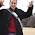## 2010-02-24

### Scala 2.8 Showcase - Collections

I would like to present some of the new features of Scala 2.8 which is a 2.8.0 Beta 1 at the moment of writing of this article.

Instead of programming dummy code I decided to implement some methods of computing square roots - but don't worry, just the simple ones. You don't have to dive in maths to understand code samples - in fact, in this article I won't really touch the square root part (I might a bit in the following articles).

This application uses a bit of Design by Contract principles and it does not use unit tests as the specs library doesn't support Scala 2.8 at the moment. Update 2010-02-26: At this moment the specs library already supports Scala 2.8. Thanks to Eric for pointing it out! You can get specs for Scala 2.8 here.

#### Scala Collections

The Scala Collections have been improved since the previous stable release.

There are three packages now, scala.collection.mutable (mutable collections), scala.collection.immutable (immutable collections), scala.collection.generic (generic collections). Almost all types of collections exist in all three forms (but not all of them; namely, there is no immutable Buffer, why would there be). The official Scala collections PDF document also presents this neat and informative diagram:

```Traversable
|
|
Iterable
+-----------------+-------------------+
|                 |                   |
Map              Set                 Seq
|                 |                   |
|             +-----+-------+         |
SortedMap    SortedSet  BitSet        |
|
|
+-----------+----------+
Buffer  Vector  LinearSeq
```

#### A bit of introduction

Now I'm going to show you a few code examples. These are nothing but simple calls of standard Scala collections methods with a bit of explanation. This is by no means a full list of methods; the list of available methods is way too long. Please refer to the official Scala collections PDF document for more information.

As I mentioned before, the program calculates the square root using two methods:
• Bisecting intervals - continuous estimations until a satisfactory result is found; for instance, when looking for a square root of 30, you can start looking for the solution between 5 (5 * 5 = 25 < 30) and 6 (6 * 6 = 36 > 30)
• Babylonian method aka Hero's method

For the example sake I provided the list of numbers to calculate square root of as a collection and then played a bit with it using Scala collections methods. Let's see some examples.

#### Creating mutable and immutable collections

```// Immutable:
val data = Set(1.0, 0.0, 1.0/4, 9.0, 16.0, 10000.0, -1.0)
```
Here we create a set using (behind the scenes) its apply (read more about the apply method on Jack Cough's blog) method. This is an immutable set because that's the one imported by default. What if we want a mutable set instead?

Well, one way is to specify the class name together with its package:
```var mutableSet = scala.collection.mutable.Set(5.0)
mutableSet += 6.0
```

The second line also demonstrates how to add elements to a mutable set; you can remove them the same way.

```mutableSet -= 6.0
println(mutableSet)
```
And it prints:
```Set(5.0)
```
BTW, what this set really is is a scala.collection.mutable.HashSet.

#### Operations

Let us now define two methods that will serve as predicates:

```def negative(x: Double) = x < 0
def equalsNumber(equalTo: Double)(x: Double) =
equalTo == x
```
The first one returns true if the given argument is less than zero. The second one is more interesting - it is a curried function. You can read more about curried functions on Daniel Spiewak's blog. Let's see them in use.

```// Immutable:
val data = Set(1.0, 0.0, 1.0/4, 9.0, 16.0, 10000.0, -1.0)
val dataOnlyPositive = data filterNot negative
println(dataOnlyPositive)
```
Here the negative method is used as a predicate given to the filter method (defined for the Iterable trait) which, as you can guess, filters the collection according to the predicate and returns the result which here is caught in a set called dataOnlyPositive. The result is:
```Set(0.0, 1.0, 0.25, 9.0, 16.0, 10000.0)
```

Let's see a few more methods:
```assert(dataOnlyPositive subsetOf data)
assert(!(data subsetOf dataOnlyPositive))

assert(dataOnlyPositive exists equalsNumber(9.0))
```

The first two calls demonstrate the subsetOf operation which checks whether the first set is a subset of the other.

The third call uses our curried function - because the exists operation accepts a predicate that expects only one parameter, we provide it one - the partial function equalsNumber(9.0). In other words, equalsNumber(9.0)(x) is itself a function that expects one argument (x). To further clarify, see the code example:

```def equalsNumber(equalTo: Double)(x: Double) =
equalTo == x

def equalsNine(x: Double) = equalsNumber(9.0)(x) // Here.
assert(!equalsNine(12))
assert(equalsNine(9))
```

#### Imports renaming

If you don't like this:

```var mutableSet = scala.collection.mutable.Set(5.0)
```

... you can always use imports renaming, a feature that is not available in Java.

```import scala.collection.mutable.{Set => MSet}
```

And now the previous call becomes:

```var mutableSet = scala.collection.mutable.Set(5.0)
```

#### Iterating over a set

One last example is iterating over a set.

```dataToProcess map {
case (x: Double) => printf(
"Calculating square root for %f.\n", x)
printf("untilInRange(0.1) condition: %.3f\n",
BisectingIntervalsMethod.approximate(x,
SatisfactionFunctions.untilInRange(
0.1)))
printf("untilInRange(0.01) condition: %.3f\n",
BisectingIntervalsMethod.approximate(x,
SatisfactionFunctions.untilInRange(
0.01)))
printf("untilInRange(0.0001)) condition: %.3f\n",
BisectingIntervalsMethod.approximate(x,
SatisfactionFunctions.untilInRange(
0.0001)))
}
```

#### Conclusion

There is a lot more to collections in Scala, so I suggest once again that you should read the official Scala collections PDF document.

#### Scala 2.8 Showcase

1. Scala 2.8 Showcase - Collections
2. Scala 2.8 Showcase - Arrays
3. Scala 2.8 Showcase - Specialized Types

1.Hi,

> as the specs library doesn't support Scala 2.8 at the moment.

Yes, it does!

You can try it out here: http://nexus.scala-tools.org/content/repositories/snapshots/org/scala-tools/testing/specs_2.8.0.Beta1/1.6.4-SNAPSHOT

Eric.

1.2.Big thanks, Eric! I tried it, it works, and I fixed the article.